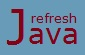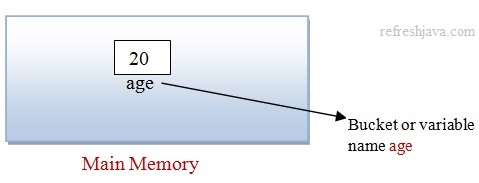# What is a variable in Java

Variables in a computer program is similar to variables in mathematics. In mathematics we use to define variable to solve a problem, similarly in computer programming we use to define variables in a program to solve a problem or accomplish a task.

Think of variable as a "Bucket" or "Envelope" where you can store information. These bucket has a name called as variable name. The information stored in a bucket can be accessed later in program by it's name. They are called variables because the information stored in it can be changed. Basic syntax of declaring a variable in java is:

` `Data_Type` variable_name = value;`

Here in place of `Data_Type` you need to specify the data type of variable, after that the name of the variable and then the variable value at right side of = operator with a semicolon(;) at the end of the declaration. For example :

`  `int` age = 20;` // variable name age with data type as int and value as 20`	`Now let's understand the different components of a variable declaration :

• Data_Type of variable - Mandatory
• Name of variable - Mandatory
• Value of variable - Optional

Each variable must have a Data Type, it can be primitive or non primitive data type. The data type of a variable represents what type of data that variable can store. For example `int` data type can store integer type values like 10, 20, 1000 etc while `float` data type can store float type values like 1.2, 10.5, 0.25 etc.

Each variable must also have a Name. The name of a variable is used to refer that variable value inside the program. The name of a variable must be unique. There are some rules and convention for writing variable names, you will get the detail about this in naming convention for identifiers tutorial.

Each variable will also have a value, either it is given by programmer or a default value is assigned by java as per it's data type. So if you declare a variable like below java will assign a default value.

`  `int` num1;`

Here as we have not assigned any value to variable `num1`, java assigns a default value which is `0` for `int` data type. Refer the data type tutorial to see the default values for each data type in java.

### What is default value of variable in Java ?

If programmer doesn't specify a value to the variable, java itself assign a value to that variable as per it's data type at runtime, this value is know as default value.

## Variable declaration and initialization in Java

Declaration and initialization of variable are two terms that is used most frequently with variables. Declaration means declaring or creating a variable while Initialization means assigning some value to that variable.

```  `int` speed;` // Declaring or creating a variable name speed`
`speed = 100;` ` // Initializing variable speed with value as 100`
`int` num1, num2;` // Declaring variable num1 and num2`
`num1 = 10; num2 = 20` ` // Initializing variable num1 and num2`
`int` count = 10; ` // Declaration and initialization together of variable count`
`double` a= 10.5, b = 20.25;` // Declaration and initialization together of variable a and b`	```

### What is difference between declaration and initialization ?

In declaration we only tell the name and the data type of the variable that we are going to create, we don't specify any value to that variable. While in initialization we provide some value to the declared variable. For example `int speed;` is a declaration while `speed = 100` is initialization.

### Variable program in Java

```` class` VariableProgram {
`public static void` main(String [] args) {
`// declaring single variable`
`int` a = 20;
`// declaring multiple variable`
`int` b = 30, c = a, d;
d = a + b;
System.out.println(`"d = "` +d);
`double` pi = 3.14159;
System.out.println(`"value of pi = "` +pi);
`boolean` e = true, f = a>c;
System.out.println(`"e = "`+e+ `"\nf = "`+f);
}
}```

Output:

d = 50
value of pi = 3.14159
e = true
f = false

Following are some of the common mistakes in declaring variables in java :

``` `int` a = 20 `// missing semicolon(;) at the end. Correct is ->  int a = 20; `
`int` b c; `// two variables must be separated by comma(,) if declared in same line. Correct is -> int b, c;`
`int` d = 20.5; `// Can't assign float value in int data type`
`int` p, `int` q; `// Correct is -> int p; int q;  or int p, q;`
`int` p = `int` q; `// Right side of = operator must be an expression or value, not variable declaration`
```

Variables can be declared at different places inside a program like inside class, methods, constructors, blocks etc. As per the declaration places, variables are divided in different types which we will see in the next tutorial.

We can also use access modifiers with variable declarations which decides the accessibility of variables in java. Refer access modifiers in java tutorial to get more detail about that.

★★★
• Always remember to add semicolon(;) at the end of variable declaration.
• To Access a variable simply means to read the information stored in that variable. To access a variable, simply type its name.
• To Assign a value to a variable means to give a value to that variable or store some value in that variable.